FreeBuf.COM网络安全行业门户，每日发布专业的安全资讯、技术剖析。FreeBuf+小程序

2018-06-06 13:00:45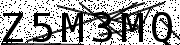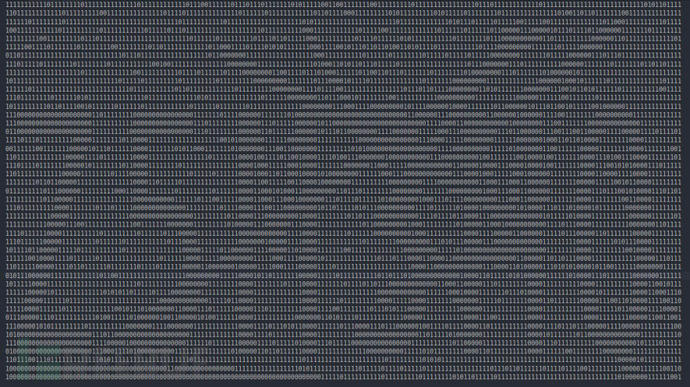``````from PIL import Image
image = Image.open("filename.xyz")
cropped_image = image.crop((left, upper, right, lower))``````

``````from PIL import Image
image = Image.open("captcha.png").convert("L") # Grayscale conversion
cropped_image = image.crop((0, 0, 30, 45))
cropped_image.save("cropped_image.png")``````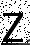``````pixel_matrix = cropped_image.load()
for col in range(0, cropped_image.height):
for row in range(0, cropped_image.width):
if pixel_matrix[row, col] != 0:
pixel_matrix[row, col] = 255
image.save("thresholded_image.png")``````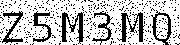``````for column in range(1, image.height - 1):
for row in range(1, image.width - 1):
if pixel_matrix[row, column] == 0 \
and pixel_matrix[row, column - 1] == 255 and pixel_matrix[row, column + 1] == 255 :
pixel_matrix[row, column] = 255
if pixel_matrix[row, column] == 0 \
and pixel_matrix[row - 1, column] == 255 and pixel_matrix[row + 1, column] == 255:
pixel_matrix[row, column] = 255``````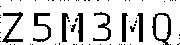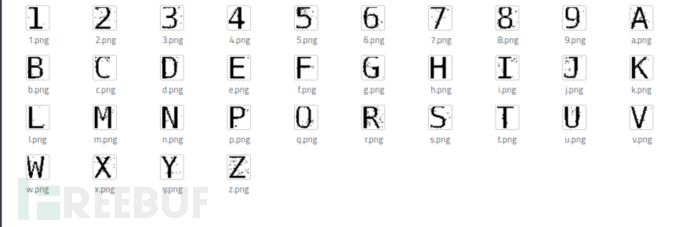``````import json
characters = "123456789abcdefghijklmnpqrstuvwxyz"
with open("bitmaps.json", "r") as f:

for j in range(image.width/6, image.width + 1, image.width/6):
character_image = image.crop((j - 30, 12, j, 44))
matches = {}
for char in characters:
match = 0
black = 0
bitmap_matrix = bitmap[char]
for y in range(0, 32):
for x in range(0, 30):
if character_matrix[x, y] == bitmap_matrix[y][x] and bitmap_matrix[y][x] == 0:
match += 1
if bitmap_matrix[y][x] == 0:
black += 1
perc = float(match) / float(black)
matches.update({perc: char.upper()})
try:
except ValueError: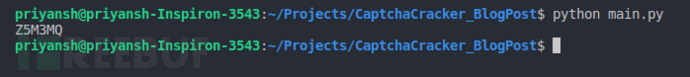## 总结

*参考来源：devFB小编 secist 编译，转载请注明来自FreeBuf.COM

# 验证码破解

+ 收入我的专辑
+ 加入我的收藏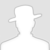\
• 0 文章数
• 0 评论数
• 0 关注者